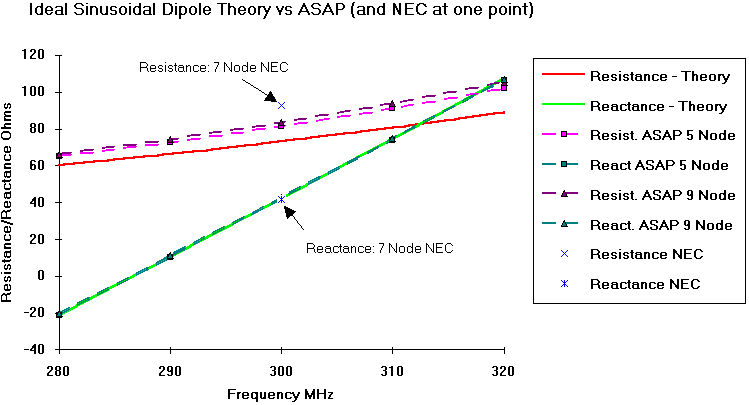# Dipole Impedance

## Numerical Accuracy

The numerical accuracy of ASAP is quite good. It should be noted that the numerical cores of most modern thin wire antenna codes are probably similar to ASAP and NEC and that the differences are usually in the input/output sophistication. In the case of ASAP sinusoidal expansion functions are used (like NEC) so only a small number of segments per wavelength are usually necessary to get good results.

The plot below illustrates the accuracy of ASAP relative to sinusoidal current theory for calculating the impedance of a dipole antenna in the vicinity of resonance. The standard textbook treatment of a wire dipole's impedance assumes that the current distribution along the length of the wire is sinusoidal. A numerical treatment such as ASAP is not restricted by this assumption.The resistance component of the antenna feedpoint impedance is larger than calculated by sinusoidal theory. This is apparently because the actual component of current that is in phase with the drive voltage is not quite sinusoidally distributed as assumed by the theory. The agreement between the reactive compent and theory is quite striking suggesting that the component of current in quadrature to the voltage is close to being sinusoidally distributed. The plot below taken from an ASAP analysis of a dipole with 10 segments illustrates this.It appears that neither component is quite sinusoidal showing that caution should be used when making use of the results of textbook "theory". The assumptions used in the textbook derivation of a result may not always match the real case. In the case of a dipole near first resonance the reactive component of impedance appears to match the numerical results quite well.

Back to: Examples Index Page

Including:

Goto Full User's Manual (from Thesis)